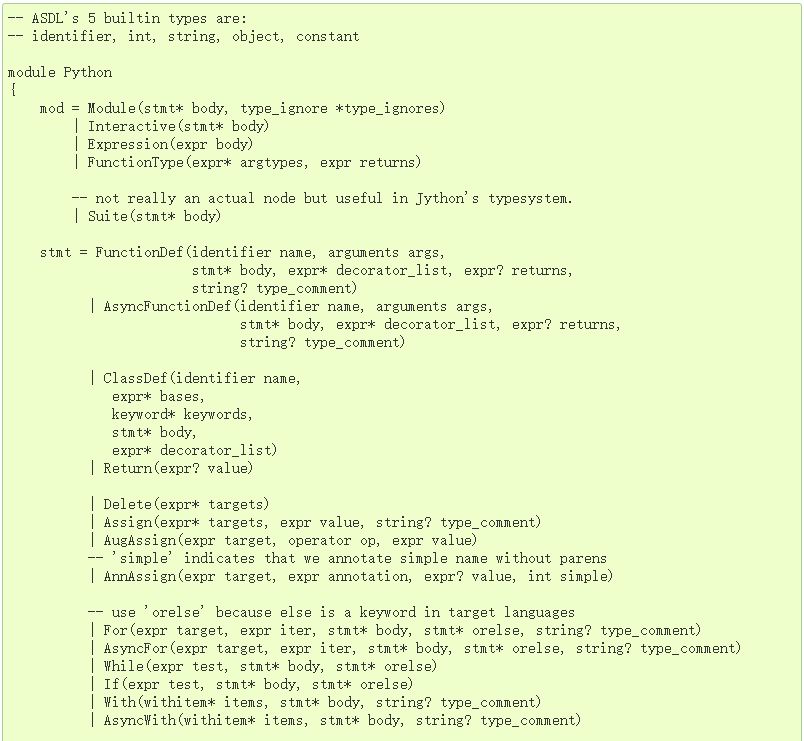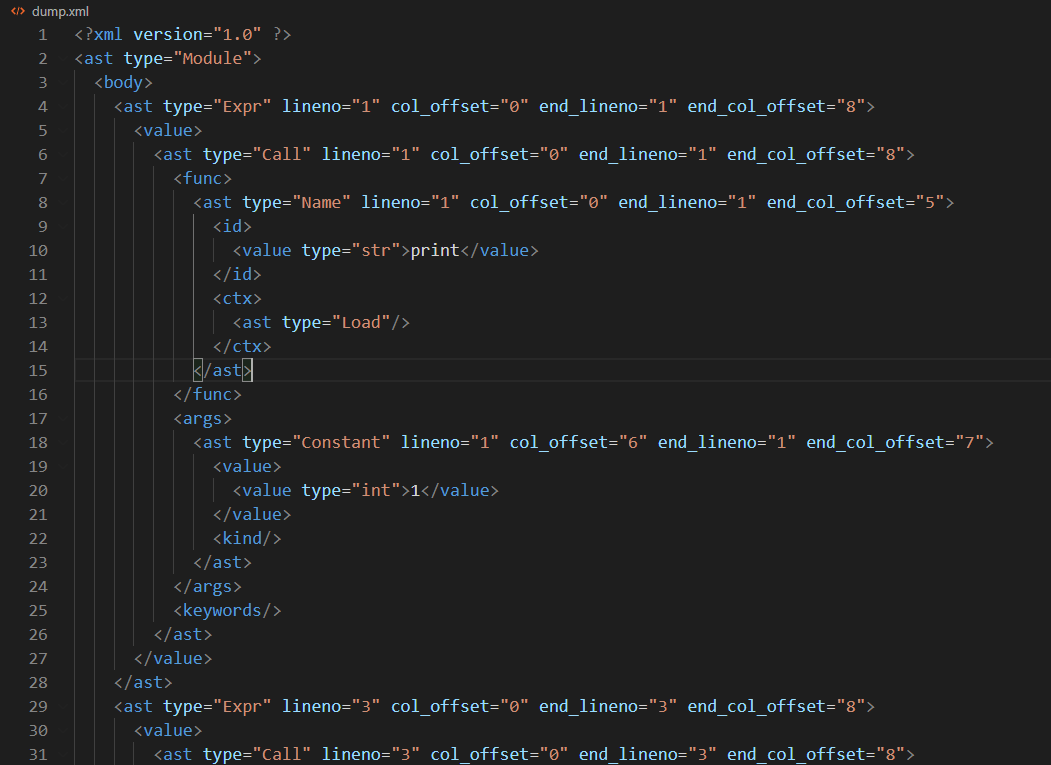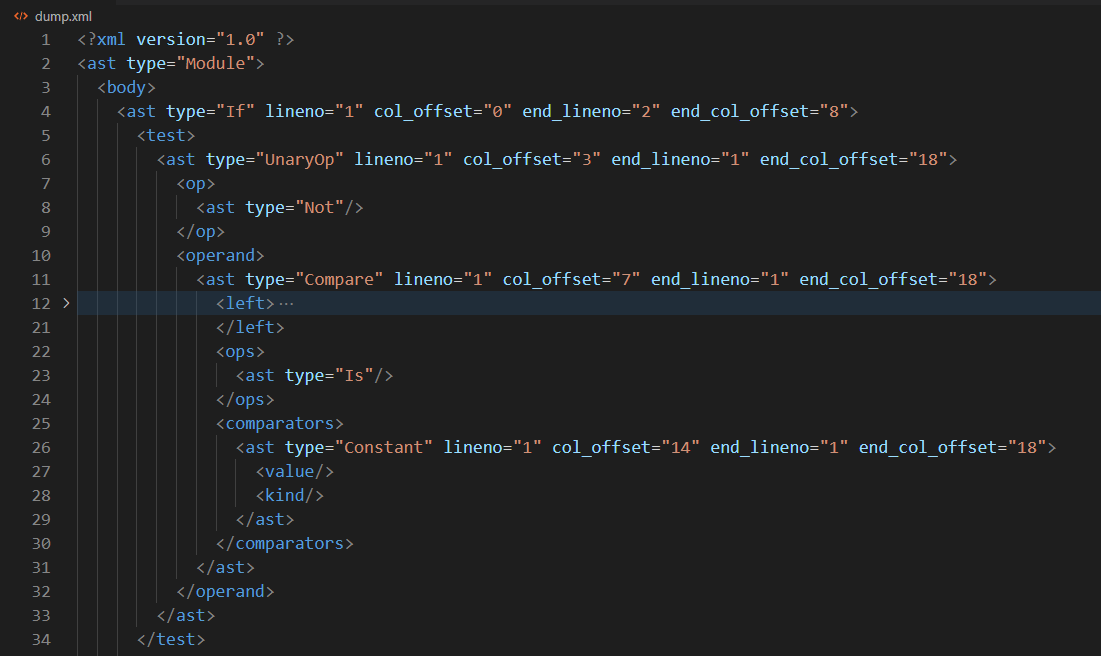# 重写 500 Lines or Less 项目 - Static Analysis

## 静态分析

`Python` 来说，从著名的 PEP8 开始，很多推荐的代码风格和指导性建议被陆续引进官方规范之中。在此基础上也诞生了众多静态分析和检查工具，比如 `pylint``pydockstyle``pyflakes``flake8`，以及各个 IDE 集成的同类功能。大家可能或多或少已经用过上述工具的其中一些了。

## `Python` 内置模块：`ast`

`ast` 模块的基本用法是相当简单的：解析源码，得到抽象语法树。

``````import ast

code = """print('Hello World!')"""
root = ast.parse(code)
print(root)
``````

``````<_ast.Module object at 0x0000021C9ECA6BE0>
``````

`Python` 程序以模块为代码组织的基本单位，因此解析到的根节点总是一个 `Module` 对象。所有节点都是 `ast.AST` 的子类。要了解完整的节点类型列表，我们可以参考官方文档 Abstract Syntax Trees:• _attributes 节点自身的属性。它们通常是一些简单的值，几乎所有节点都包括以下四个属性：`lineno`/`col_offset`/`end_lineno`/`end_col_offset`。显然，它们表示元素在代码中的起止位置。有点不太直观的是，`lineno` 是从 1 开始计数，而 `col_offset` 却是从 0 开始计数的。这是设计上一点缺乏一致性的地方。
• _fields 包含节点持有的“子节点”。这是一个有点抽象的说法，实际上它可能是以下三种情况之一：

• 节点本身持有的信息，通常是比较复杂的对象

• 另一个 `AST` 节点，也就是该节点的子节点
• 在更复杂的情况下可能是列表（`list`），表示多个子节点的集合。

`NodeVisitor` 是一个抽象基类。要使用它，我们需要继承它得到一个派生类，再按照自己的要求添加成员。常见的实现模式有以下两种：

• 如果我们关心某种特定类型的的节点，按照 `NodeVisitor` 的约定，需要添加一个名为 `visit_XXXX` 的方法，其名称和节点的类名严格对应（各种节点对应的类型可参考前面给出的 `AST` 文档），参数是访问到的节点。例如，要访问函数定义，则添加方法 `visit_FunctionDef(node)`，依此类推；
• 如果想要同时支持多种节点类型，或有其他特殊要求，可以重载其 `visit(node)` 方法。

``````class MyVisitor(ast.NodeVisitor):
def visit(self, node: ast.AST):
print('visit node:', node)
return self.generic_visit(node)

def visit_FunctionDef(self, node: ast.FunctionDef):
print('visit function:', node)
return self.generic_visit(node)
``````

``````class NodeVisitor(object):
def visit(self, node):
method = 'visit_' + node.__class__.__name__
visitor = getattr(self, method, self.generic_visit)
return visitor(node)

def generic_visit(self, node):
for field, value in iter_fields(node):
if isinstance(value, list):
for item in value:
if isinstance(item, AST):
self.visit(item)
elif isinstance(value, AST):
self.visit(value)
``````

## 输出 AST

``````Module(body=[FunctionDef(name='add', args=arguments(posonlyargs=[], args=[arg(arg='a', annotation=None, type_comment=None), arg(arg='b', annotation=None, type_comment=None)], vararg=None, kwonlyargs=[], kw_defaults=[], kwarg=None, defaults=[]), body=[Return(value=BinOp(left=Name(id='a', ctx=Load()), op=Add(), right=Name(id='b', ctx=Load())))], decorator_list=[], returns=None, type_comment=None)], type_ignores=[])
``````

• 首先，AST 的复杂性可能会超出你的预料。输出到普通文本，哪怕是带有缩进的文本，其内容仍然过于复杂。而 XML 可以很好地展示内部结构；
• 其次，现代编辑器对 XML 这样的结构化文本都有完善的支持，用户可以展开或折叠特定地节点，逐步了解各个层次结构，同时忽略那些不需要关心的部分；
• 有的朋友可能会问，为什么不使用 `JSON`？网络上很多资料会告诉你 `JSON``XML` 更简单，但那是有条件的。对于 AST 这种复杂的结构，`XML` 每个元素都有明确的名字，可以清楚地看到每个节点的类型，而 `JSON` 必须付出额外的努力，才能理解每一层大括号到底表示什么含义。另外，`JSON` 文档格式化以后太过冗长，同时嵌套层次又非常深，对于阅读来说其实是非常不利的。使用 `XML` 还有一个好处，那就是 `AST``_attributes``_fields` 恰好能匹配到 XML 中 `Attribute``Element` 的概念，所以处理起来更加自然。

``````import ast
from astxml import AstXml

code = """
return a + b
""".strip()

ast_node = ast.parse(source_code)
AstXml(ast_node).save_file('filename.xml')
``````• AST 的根节点总是从 `Module` 开始；
• 除了 `Module` 之外，所有 AST 节点几乎都包含起止位置的行号/列号，也就是 `lineno/col_offset/end_lineno/end_col_offset` 这几个字段；
• 每种 Python 语法有自己的 AST 结构。比如 `print()` 方法调用，我们可以再分析其结构：
• 它是一个表达式（`Expr`
• 表达式内容是函数调用（`Call`
• 函数引用是一个 `Name``Name.id` 记录其具体名称
• 参数是一个集合，对于常量参数，表示为 `Constant`

## 编写程序

### 数据结构设计

``````@dataclass
class CodeIssue:
filename: str = None
line: int = 0
column: int = 0
code: str = None
message: str = None

def __str__(self):
pattern = '{file}({line},{col}) {code}: {message}'
return pattern.format(file=self.filename,
line=self.line,
col=self.column,
code=self.code,
message=self.message)
``````

``````class AnalysisContext:
def __init__(self, filename: str):
self.filename = filename
self.issues = []

def add_issue(self, node: ast.AST, code: str, message: str):
issue = CodeIssue(filename=self.filename,
line=node.lineno,
column=node.col_offset,
code=code,
message=message)
self.issues.append(issue)
``````

### 代码长度检查

`PEP8` 中对代码长度有这样的要求：

Limit all lines to a maximum of 79 characters.

For flowing long blocks of text with fewer structural restrictions (docstrings or comments), the line length should be limited to 72 characters.

``````class LineLengthVisitorTest(TestCase, VisitorTestMixin):
visitor_type = LineLengthVisitor

def test_visit(self):
code = """
print('short line')
print('this is a very, very, very, very, very, very, very, very, very, very, very, very long line...')
""".strip()
ctx = AnalysisContext('test.py')
visitor = LineLengthVisitor(self.ctx)
ast_node = ast.parse(code)
visitor.visit(ast_node)
AstXml(ast_node).save_file('dump/line-length.xml')
self.assertEqual(1, len(ctx.issues))
issue = ctx.issues
self.assertEqual((2, 'W0001'), (issue.line, issue.code))
``````

``````class LineLengthVisitor(ast.NodeVisitor):
max_length = 79

def __init__(self, ctx: AnalysisContext):
super().__init__()
self.ctx = ctx

def visit(self, node: ast.AST):
if 'end_col_offset' in node._attributes and node.end_col_offset > self.max_length:
self.ctx.add_issue(node, 'W0001', f'Exceed max line length({self.max_length})')
else:
self.generic_visit(node)
``````

For flowing long blocks of text with fewer structural restrictions (docstrings or comments), the line length should be limited to 72 characters.

``````    def test_visit_docstring(self):
code = """
def fn():
'''
This is a very, very, very, very, very, very, very, very, very, very, very, very long doc string
The second line
'''
pass
""".strip()
...
issue = ctx.issues
self.assertEqual((3, 'W0001'), (issue.line, issue.code))
``````

``````    def get_docstring_node(self, node):
"""Return the AST node of docstring"""
if not isinstance(node, (ast.AsyncFunctionDef, ast.FunctionDef, ast.ClassDef, ast.Module)):
raise TypeError("%r can't have docstrings" % node.__class__.__name__)
if not(node.body and isinstance(node.body, ast.Expr)):
return None
value_node = node.body.value
if isinstance(value_node, ast.Constant) and isinstance(value_node.value, str):
return value_node
return None
``````

``````class LineLengthVisitor(BaseVisitor):
max_length = 79
max_docstring_length = 72

def visit(self, node: ast.AST):
if isinstance(node, ast.FunctionDef):
self.check_docstring(node)
# ... origin code

def check_docstring(self, node: ast.AST):
doc_node = self.get_docstring_node(node)
if not doc_node:
return self.generic_visit(node)
for offset, line in enumerate(doc_node.value.split('\n')):
if len(line) >= self.max_docstring_length:
lineno = doc_node.lineno + offset
f'Docstring for {node.name} exceed max length({self.max_docstring_length})',
lineno=lineno)
``````

``````class VisitorTestMixin:
visitor_type = None

def run_visitor(self, code: str, xml_filename: str = None):
self.assertIsNotNone(self.visitor_type, 'Visitor type not defined.')
self.ctx = AnalysisContext('test.py')
visitor = self.visitor_type(self.ctx)
ast_node = ast.parse(code)
visitor.visit(ast_node)
if xml_filename:
AstXml(ast_node).save_file('dump/' + xml_filename)
``````

``````class LineLengthVisitorTest(TestCase, VisitorTestMixin):
visitor_type = LineLengthVisitor

def test_visit(self):
code = ...
self.run_visitor(code, xml_filename='line-length.xml')
self.assert_found_issue(2, 'W0001')
``````

### 异常类型检查

``````# Correct
try:
x = my_dict[key]
except KeyError:
...

# Wrong
try:
x = my_dict[key]
except Exception:
# or except:
...
``````• 同时捕获多个异常类型，如 `except (ValueError, KeyError)`
• 不指定具体类型，只包含一个简单的 `except`

``````class ExceptionTypeVisitorTest(TestCase, VisitorTestMixin):
visitor_type = ExceptionTypeVisitor

def test_no_handler(self):
code = """print('hello')""".strip()
self.run_visitor(code, xml_filename='exception-no-handler.xml')
self.assert_no_issue()

def test_handler_generic(self):
code = """
try:
calc()
except Exception as e:
print(e)
""".strip()
self.run_visitor(code, xml_filename='exception-catch-generic.xml')
self.assert_found_issue(3, 'W0002')
``````

``````class ExceptionTypeVisitor(BaseVisitor):
def visit_ExceptHandler(self, node: ast.ExceptHandler):
exp_type = node.type
if isinstance(exp_type, ast.Name) and exp_type.id == 'Exception':
self.ctx.add_issue(node, 'W0002', f'Avoid catch generic Exception.')
self.generic_visit(node)
``````

``````    def test_catch_multiple_types_with_issue(self):
code = """
try:
calc()
except (Exception, ValueError) as e:
print(e)
""".strip()
self.run_visitor(code, xml_filename='exception-catch-multi-with-issue.xml')
self.assert_found_issue(3, 'W0002')
``````

``````class ExceptionTypeVisitor(BaseVisitor):
AVOID_TYPES = ('Exception', 'BaseException')

def iter_exception_types(self, node: ast.AST):
if isinstance(node, ast.Name):
yield node
elif isinstance(node, ast.Tuple):
for name_node in node.elts:
if isinstance(name_node, ast.Name):
yield name_node

def visit_ExceptHandler(self, node: ast.ExceptHandler):
for name_node in self.iter_exception_types(node.type):
if name_node.id in self.AVOID_TYPES:
self.ctx.add_issue(name_node, 'W0002', f'Avoid catch generic Exception.')
self.generic_visit(node)
``````

``````    def test_catch_no_type(self):
code = """
try:
calc()
except:
print(e)
""".strip()
self.run_visitor(code, xml_filename='exception-catch-no-type.xml')
self.assert_found_issue(3, 'W0002')
``````

``````    def visit_ExceptHandler(self, node: ast.ExceptHandler):
if not node.type:
# ... origin code
``````

### 变量使用检查

``````class VariableUsageVisitorTest(TestCase, VisitorTestMixin):
visitor_type = VariableUsageVisitor

def test_vars_all_used(self):
code = """
def fn():
name = 'user'
print(name)
""".strip()
self.run_visitor(code, xml_filename='var-used.xml')
self.assert_no_issue()

def test_vars_not_used(self):
code = """
def fn():
name = 'user'
print('hello')
""".strip()
self.run_visitor(code, xml_filename='vars-unused.xml')
self.assert_found_issue(2, 'W0003')
``````

``````class VariableUsageVisitorTest(TestCase, VisitorTestMixin):
visitor_type = VariableUsageVisitor

def test_no_func(self):
code = """print('hello')""".strip()
self.run_visitor(code, xml_filename='var-no-func.xml')
self.assert_no_issue()

def test_global_vars_used(self):
code = """
name = 'user'
print(name)
""".strip()
self.run_visitor(code, xml_filename='global-vars-used.xml')
self.assert_no_issue()

def test_global_vars_unused(self):
code = """
name = 'user'
print('hello')
""".strip()
self.run_visitor(code, xml_filename='global-vars-unused.xml')
self.assert_found_issue(1, 'W0003')
``````

``````class VariableUsageVisitor(BaseVisitor):
def __init__(self, ctx: AnalysisContext):
super().__init__(ctx)
self.in_assign = False
self.declare_vars = {}
self.used_vars = set()

def visit_Assign(self, node: ast.Assign):
self.in_assign = True
self.generic_visit(node)
self.in_assign = False
``````

``````    def visit_Name(self, node: ast.Name):
var_name = node.id
if self.in_assign:
self.declare_vars[var_name] = node
else:
``````

``````    def visit_Module(self, node: ast.Module):
self.generic_visit(node)
unused_vars = set(self.declare_vars) - self.used_vars
for var_name in unused_vars:
node = self.declare_vars[var_name]
self.ctx.add_issue(node, 'W0003', f"Variable '{var_name}' declared but never used")
``````

``````    def test_nested_funcs(self):
code = """
def outer():
def inner():
name = 'inner'
print('hello')
name = 'outer'
print(name)
""".strip()
self.run_visitor(code, xml_filename='vars-nested-func.xml')
self.assert_found_issue(3, 'W0003')
``````

`VariableUsageVisitor` 的实现也需要做比较大的修改。我们在其构造函数中增加一个列表，用来记录作用域的堆栈：

``````class VariableUsageVisitor(BaseVisitor):
def __init__(self, ctx: AnalysisContext):
super().__init__(ctx)
self.in_assign = False
self.scope_stack = []
``````

``````    def visit(self, node: ast.AST):
is_scope_ast = isinstance(node,
(ast.Module, ast.FunctionDef, ast.ClassDef))
if is_scope_ast:
scope = VariableScope(node)
self.scope_stack.append(scope)
super().visit(node)
if is_scope_ast:
self.scope_stack.remove(scope)
scope.check(self.ctx)
``````

``````    def visit_Name(self, node: ast.Name):
if self.scope_stack:
scope = self.scope_stack[-1]
scope.use(node, self.in_assign)
self.generic_visit(node)
``````

### 语序问题检查

`Python` 以简洁易懂而著称，也特别重视语句是否直观、自然。比如，以下两种写法在逻辑上是等效的，但 `PEP8` 明确指出：应该尽可能地使用第一种写法。

``````# Correct
if foo is not None: pass
# Wrong
if not foo is None: pass
````````````class PreferIsNotVisitorTest(TestCase, VisitorTestMixin):
visitor_type = PreferIsNotVisitor

def test_is_not(self):
code = """
if a is not None:
print(a)
""".strip()
self.run_visitor(code, xml_filename='is-not.xml')
self.assert_no_issue()

def test_not_is(self):
code = """
if not a is None:
print(a)
""".strip()
self.run_visitor(code, xml_filename='not-is.xml')
self.assert_found_issue(1, 'W0004')
``````

``````class PreferIsNotVisitor(BaseVisitor):
def visit_If(self, node: ast.If):
if isinstance(node.test, ast.UnaryOp) and \
isinstance(node.test.op, ast.Not):
operand = node.test.operand
if isinstance(operand, ast.Compare) and \
len(operand.ops) == 1 and \
isinstance(operand.ops, ast.Is):
self.generic_visit(node)
``````

### 汇总

``````class CodeAnalyzer:
def visitors(self, ctx: AnalysisContext):
yield LineLengthVisitor(ctx)
yield ExceptionTypeVisitor(ctx)
yield VariableUsageVisitor(ctx)
yield PreferIsNotVisitor(ctx)

def analysis(self, filename: str, code: str):
self.ctx = AnalysisContext(filename)
ast_root = ast.parse(code)
for visitor in self.visitors(self.ctx):
visitor.visit(ast_root)

def print(self):
for issue in self.ctx.issues:
print(issue)

if __name__ == '__main__':
analyzer = CodeAnalyzer()
analyzer.analysis('test.py', CODE)
analyzer.print()
``````

`CodeAnalyzer` 使用所有支持的 `NodeVisitor` 来访问程序，并记录检查结果。如果以后实现了新的 `visitor`，只要简单地把它加入 `visitors` 集合即可。

## 文章结构

• 基本原理和工具

• 实现加法

• 函数调用 在上一步的基础上实现调用 Python 内部函数的功能，以扩展解释器的应用范围；

• 分支判断

`if` 语句为例，实现分支和跳转指令。该步骤需要对解释器的执行过程进行重构；

• 自定义函数

• 列表解析

## 理解 Python 字节码``````>>> def f(): return 1
...
>>> f.__code__
<code object f at 0x00000171E2253F50, file "<stdin>", line 1>
>>> f.__code__.co_code
b'd\x01S\x00'
``````

``````import dis

def main():
print("====dis code====")
source = "n = a + 1"
code = compile(source, filename='', mode='exec')
print('co_names:', code.co_names)
print('co_consts:', code.co_consts)
print('co_code', code.co_code)
dis.dis(code)

print("====instructions====")
for instruction in dis.get_instructions(code):
print(instruction.opcode, instruction.opname, instruction.arg, instruction.offset)
``````

``````====dis code====
co_names: ('a', 'n')
co_consts: (1, None)
co_code b'e\x00d\x00\x17\x00Z\x01d\x01S\x00'
6 STORE_NAME               1 (n)
10 RETURN_VALUE
====instructions====
90 STORE_NAME 1 6
83 RETURN_VALUE None 10
``````

`dis` 以列表的形式显示字节码指令，不过它的格式需要一点时间来熟悉。一般来说，这个列表会有 5 列，从左到右，它们的内容包括：

• 对应源码的行号
• 指令在整个字节码中的偏移量
• 指令名称
• 指令的参数（如果有的话）
• 对指令参数的含义进行补充说明，以便于理解。比如上面字节码的第一条指令 `LOAD_NAME` 参数为 0， 表示需要取 `co_names` 也就是变量 a 的内容作为值。

STORE_NAME(1) 将结果记录到 co_names 对应的变量，也就是 n
RETURN_VALUE 返回值

• `LOAD_NAME` 取出一个变量，并压入堆栈；
• `LOAD_CONST` 取出一个常量，也压入堆栈；
• 现在堆栈上有两个值，`BINARY_ADD` 指令从栈上弹出两个参数，执行加法计算，把结果再次压入堆栈；
• `STORE_NAME` 从堆栈上取得计算结果，并保存到变量 n。
• 字节码的最后一个指令通常都是 `RETURN_VALUE`。但我们目前还没有涉及到函数调用，模块级别的返回值并不重要，因此可以暂时忽略它。

## 基本运算

``````import unittest
from .interpreter import Interpreter

class InterpreterTest(unittest.TestCase):
source = "n = a + 1"
interpreter = Interpreter(source)
interpreter.set_local('a', 2)
interpreter.exec()
self.assertEqual(3, interpreter.get_local('n'))
``````

1. `dis` 模块将代码翻译成指令
2. 将执行所需的常量和变量等注入执行环境
3. 依次执行各条指令

``````import dis

class Interpreter:
def __init__(self, source):
self._code = compile(source, filename='', mode='exec')
self._locals = {}

def get_local(self, name):
return self._locals[name]

def set_local(self, name, value):
self._locals[name] = value

def get_const(self, consti):
return self._code.co_consts[consti]
``````

``````class Interpreter:
def __init__(self, source):
...
self._stack = []

def stack_push(self, value):
self._stack.append(value)

def stack_pop(self):
return self._stack.pop(-1)
``````

``````    def exec(self):
for instruction in dis.get_instructions(self._code):
fn = getattr(self, 'exec_' + instruction.opname)
fn(instruction.arg)

...
``````

``````LOAD_NAME(namei)
Pushes the value associated with co_names[namei] onto the stack.
``````

``````    def exec_LOAD_NAME(self, namei):
name = self.get_name(namei)
value = self.get_local(name)
self.stack_push(value)
``````

`BINARY_ADD` 则需要从栈上弹出两个操作数，相加后再次放到栈上：

``````    def exec_BINARY_ADD(self, _):
tos = self.stack_pop()
tos1 = self.stack_pop()
result = tos1 + tos
self.stack_push(result)
``````

``````    def exec_RETURN_VALUE(self, _):
pass
``````

``````    def dump_code(self):
print(f"====dis code of {self._code.co_name}====")
print('co_names:', self._code.co_names)
print('co_consts:', self._code.co_consts)
print('co_code', self._code.co_code)
print('co_varnames', self._code.co_varnames)
dis.dis(self._code)

def dump_stack(self, instruction):
print(f'Stack after {instruction.opname}({instruction.offset}): {self._stack}')
``````

## 调用函数

``````class InterpreterTest(unittest.TestCase):
def exec_interpreter(self, source, local_vars=None, dump_code=False, trace_stack=False):
interpreter = Interpreter(source,
local_vars=local_vars,
dump_code=dump_code, trace_stack=trace_stack)
interpreter.exec()
return interpreter

...

def test_call_func(self):
source = "n = divmod(a, 2)"
interpreter = self.exec_interpreter(source, {'a': 11}, False, False)
self.assertEqual((5, 1), interpreter.get_local('n'))
``````

``````====dis code of <module>====
co_names: ('divmod', 'a', 'n')
co_consts: (2, None)
co_code b'e\x00e\x01d\x00\x83\x02Z\x02d\x01S\x00'
co_varnames ()
6 CALL_FUNCTION            2
8 STORE_NAME               2 (n)
12 RETURN_VALUE
``````

``````>>> import builtins
>>> getattr(builtins, 'divmod')
``````

``````import builtins

class Interpreter:
def __init__(self, source, local_vars=None, dump_code=False, trace_stack=False):
...
self._builtins = {x: getattr(builtins, x) for x in dir(builtins) if not x.startswith('__')}

name = self.get_name(namei)
if name in self._locals:
value = self.get_local(name)
elif name in self._builtins:
value = self._builtins[name]
else:
raise NameError(name)
self.stack_push(value)
``````

``````    def stack_popn(self, count):
if count > 0:
result = self._stack[-count:]
self._stack = self._stack[:-count]
return result
return []

def exec_CALL_FUNCTION(self, argc):
args = self.stack_popn(argc)
func = self._stack.pop(-1)
result = func(*args)
self.stack_push(result)
``````

## 实现 if 分支

``````    def test_if(self):
source = """
if a > 10:
b = True
else:
b = False
""".strip()
interpreter = self.exec_interpreter(source, {'a': 11}, True, True)
self.assertEqual(True, interpreter.get_local('b'))

interpreter = self.exec_interpreter(source, {'a': 3}, False, False)
self.assertEqual(False, interpreter.get_local('b'))
``````

``````co_names: ('a', 'b')
co_consts: (10, True, False, None)
co_code b'e\x00d\x00k\x04r\x0ed\x01Z\x01n\x04d\x02Z\x01d\x03S\x00'
4 COMPARE_OP               4 (>)
6 POP_JUMP_IF_FALSE       14

10 STORE_NAME               1 (b)
12 JUMP_FORWARD             4 (to 18)

4     >>   14 LOAD_CONST               2 (False)
16 STORE_NAME               1 (b)
20 RETURN_VALUE```
``````

### COMPARE_OP

``````>>> dis.cmp_op
('<', '<=', '==', '!=', '>', '>=', 'in', 'not in', 'is', 'is not', 'exception match', 'BAD')
``````

### JUMP_FORWARD

• `POP_JUMP_IF_FALSE` 指令的地方开始代码分支，如果值为 True 则执行位置 8~12 部分的代码，否则执行 14~18 部分；
• 8~12 部分执行完毕后，通过 `JUMP_FORWARD` 指令跳转到最后；
• 14~16 部分正常执行到最后。

``````class ReturnValue(Exception):
pass

class Interpreter:
def __init__(self, source):
...
self._instructions = list(dis.get_instructions(self._code))
self._next_instruction = 0

def exec(self):
while True:
try:
instruction = self._instructions[self._next_instruction]
fn = getattr(self, 'exec_' + instruction.opname)
if not fn(instruction.arg):
self._next_instruction += 1
except ReturnValue:
break

def exec_RETURN_VALUE(self, _):
raise ReturnValue()
``````

``````    def exec_COMPARE_OP(self, opname):
opname = dis.cmp_op[opname]
comparers = {
'<': lambda x, y: x < y,
'<=': lambda x, y: x <= y,
'==': lambda x, y: x == y,
'!=': lambda x, y: x != y,
'>': lambda x, y: x > y,
'>=': lambda x, y: x >= y,
'in': lambda x, y: x in y,
'not in': lambda x, y: x not in y,
'is': lambda x, y: x is y,
'is not': lambda x, y: x is not y,
}
comparer = comparers[opname]
rhs = self.stack_pop()
lhs = self.stack_pop()
result = comparer(lhs, rhs)
self.stack_push(result)
``````

`POP_JUMP_IF_FALSE` 略微复杂，因为要处理字节码偏移量的问题。后面会看到，“按偏移量跳转”对于大多数跳转指令而言是常规操作，因此我们把它封装为辅助方法：

``````    def exec_POP_JUMP_IF_FALSE(self, target):
value = self.stack_pop()
if not value:
self.jump_by_offset(target)
return True
return False

def jump_by_offset(self, offset):
index = [index for index, instruction in enumerate(self._instructions)
if instruction.offset == offset]
self._next_instruction = index
``````

``````    def exec_JUMP_FORWARD(self, delta):
offset = self._instructions[self._next_instruction + 1].offset + delta
self.jump_by_offset(offset)
return True
``````

## 定义函数

``````    def test_define_func(self):
source = """
def f(x):
return x + 1

n = f(a)
""".strip()
interpreter = self.exec_interpreter(source, {'a': 11}, True, True)
self.assertEqual(12, interpreter.get_local('n'))
``````

``````====dis code of <module>====
co_names: ('f', 'a', 'n')
co_consts: (<code object f at 0x00000257F23D83A0, file "", line 1>, 'f', None)
co_code b'd\x00d\x01\x84\x00Z\x00e\x00e\x01\x83\x01Z\x02d\x02S\x00'
co_varnames: ()
1           0 LOAD_CONST               0 (<code object f at 0x00000257F23D83A0, file "", line 1>)
4 MAKE_FUNCTION            0
6 STORE_NAME               0 (f)

12 CALL_FUNCTION            1
14 STORE_NAME               2 (n)
18 RETURN_VALUE

Disassembly of <code object f at 0x00000257F23D83A0, file "", line 1>:
6 RETURN_VALUE
``````

• 函数创建一个运行时结构，在 Python 解释器的术语中称为“Frame”，表示一个独立的执行空间，有它自己的作用域、指令和堆栈；
• Frame 也是用一个类似堆栈的运行时结构来管理的。每次调用函数都会形成一个新的 Frame，并放到堆栈的顶部，执行完毕后从栈中清除；
• Frame 可以“看到”调用它的那个对象（可能是主模块，也可能是另一个函数）定义的数据，并且可以层层上溯直到全局变量（这就是 Python 查找变量的规则）；
• 当 Frame 执行时，它所需要的参数从调用者空间拷贝到被调用者的执行空间（每个 Frame 有自己的执行堆栈）；
• Frame 依次执行指令（以及跳转），一旦遇到 RETURN_VALUE 指令，则该 Frame 执行完毕，该 Frame 将会被清除，但返回值会传递给被调用者
• 被调用者从函数调用后的代码继续执行

``````def dump_recursive(code):

def dump(acode):
print(f"====dis code of {acode.co_name}====")
print('co_names:', acode.co_names)
print('co_consts:', acode.co_consts)
print('co_code', acode.co_code)
print('co_varnames:', acode.co_varnames)
dis.dis(acode, depth=0)

dump(code)
for item in code.co_consts:
if hasattr(item, 'co_code'):
dump_recursive(item)
``````

``````====dis code of f====
co_names: ()
co_consts: (None, 1)
co_varnames: ('x',)
co_code b'|\x00d\x01\x17\x00S\x00'
6 RETURN_VALUE
``````

``````class Frame:
def __init__(self, interpreter, code, scope):
self._interpreter = interpreter
self._code = code
self._instructions = list(dis.get_instructions(self._code))
self._next_instruction = 0
self.scope = scope.new_child()
self._stack = []

def get_local(self, name):
return self.scope[name]

def set_local(self, name, value):
self.scope[name] = value

...
``````

``````class Interpreter:
def __init__(self, source, local_vars=None,
dump_code=False, trace_stack=False):
self._code = compile(source, filename='', mode='exec')
self._dump_code = dump_code
self.trace_stack = trace_stack
builtin_dict = {x: getattr(builtins, x) for x in dir(builtins) if not x.startswith('__')}
self._scope = ChainMap(builtin_dict)
self._frames = []

main_frame = Frame(self, self._code, self._scope)
if local_vars:
for k, v in local_vars.items():
main_frame.set_local(k, v)
self._frames.append(main_frame)
# main frame treat as global scope and never pop

def top_frame(self):
return self._frames[-1]

def get_local(self, name):
return self.top_frame().get_local(name)

def set_local(self, name, value):
self.top_frame().set_local(name, value)

def frame_push(self, frame):
self._frames.append(frame)

def frame_pop(self):
if len(self._frames) == 1:
raise RuntimeError('main frame cannot pop out')
return self._frames.pop(-1)

def exec(self):
if self._dump_code:
dump_recursive(self._code)
self.top_frame().exec()
``````

`_frames` 也是一个类似于堆栈的结构，每次调用函数就会形成一个新的 Frame，而执行完毕后就会被清除。解释器一开始就会初始化主模块，但执行完毕后并不从栈上弹出——因为我们还要检查主模块的变量值。为了避免出错，`frame_pop()` 部分包含了额外的检查。

``````    def exec_MAKE_FUNCTION(self, flags):
name = self.stack_pop()
code = self.stack_pop()
freevars, annonations, defaults, kwdefaults = None, None, None, None
if flags & 0x8:
freevars = self.stack_pop()
if flags & 0x4:
annonations = self.stack_pop()
if flags & 0x2:
kwdefaults = self.stack_pop()
if flags & 0x1:
defaults = self.stack_pop()
func = Function(self._interpreter, name, code, freevars, annonations, kwdefaults, defaults)
self.stack_push(func)
``````

`MAKE_FUNCTION` 只是定义了函数，它会被后续的指令保存在局部变量中。只有真正执行（也就是`CALL_FUNCTION`）的时候它才会真正构造出一个 Frame。

• 调用内置函数。这时我们只需要像普通代码一样执行它——运行时会处理所有细节
• 调用自定义函数：我们需要自己处理参数压栈/出栈的操作

``````class Function:
def __init__(self, interpreter, name, code, freevars, annonations, kwdefaults, defaults):
self.interpreter = interpreter
self.name = name
self.code = code
self.freevars = freevars
self.annonations = annonations
self.kwdefaults = kwdefaults
self.defaults = defaults

def __call__(self, *args, **kwargs):
frame = Frame(self.interpreter, self.code, self.interpreter.top_frame().scope)
frame.set_args(args)
self.interpreter.frame_push(frame)
result = frame.exec()
self.interpreter.frame_pop()
return result
``````

``````    def set_args(self, args):
for varnum, arg in enumerate(args):
name = self._code.co_varnames[varnum]
self.set_local(name, arg)
``````

``````    def exec_LOAD_FAST(self, varnum):
name = self._code.co_varnames[varnum]
value = self.get_local(name)
self.stack_push(value)
``````

``````class ReturnValue(Exception):
def __init__(self, value):
self.value = value

class Frame:
def exec_RETURN_VALUE(self, _):
value = self.stack_pop()
raise ReturnValue(value)

def exec(self):
while True:
try:
...
except ReturnValue as e:
return e.value
``````

## 列表解析

``````    def test_list_comprehension(self):
source = """
n = [x for x in range(a) if x>5]
""".strip()
interpreter = self.exec_interpreter(source, {'a': 10}, True, True)
self.assertEqual([6, 7, 8, 9], interpreter.get_local('n'))
``````

``````====dis code of <module>====
co_names: ('range', 'a', 'n')
co_consts: (<code object <listcomp> at 0x0000017C38C6DB30, file "", line 1>, '<listcomp>', None)
co_code: b'd\x00d\x01\x84\x00e\x00e\x01\x83\x01D\x00\x83\x01Z\x02d\x02S\x00'
co_varnames: ()
1           0 LOAD_CONST               0 (<code object <listcomp> at 0x0000017C38C6DB30, file "", line 1>)
4 MAKE_FUNCTION            0
10 CALL_FUNCTION            1
12 GET_ITER
14 CALL_FUNCTION            1
16 STORE_NAME               2 (n)
20 RETURN_VALUE
====dis code of <listcomp>====
co_names: ()
co_consts: (5,)
co_code: b'g\x00|\x00]\x10}\x01|\x01d\x00k\x04r\x04|\x01\x91\x02q\x04S\x00'
co_varnames: ('.0', 'x')
1           0 BUILD_LIST               0
>>    4 FOR_ITER                16 (to 22)
6 STORE_FAST               1 (x)
12 COMPARE_OP               4 (>)
14 POP_JUMP_IF_FALSE        4
18 LIST_APPEND              2
20 JUMP_ABSOLUTE            4
>>   22 RETURN_VALUE
``````

• `BUILD_LIST(n)`

• `FOR_ITER(delta)`

• `LIST_APPEND(i)`

• `JUMP_ABSOLUTION(taret)`

``````    def exec_BUILD_LIST(self, count):
value = self.stack_popn(count)
self.stack_push(value)

def exec_FOR_ITER(self, delta):
it = self.stack_top()
try:
value = next(it)
self.stack_push(value)
except StopIteration:
self.stack_pop()
self.jump_delta(delta)
return True

def exec_LIST_APPEND(self, i):
value = self.stack_pop()
l = self._stack[-i]
assert isinstance(l, list)
l.append(value)

def exec_JUMP_ABSOLUTE(self, target):
self.jump_by_offset(target)
return True
``````